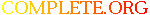Complete.Org: Mailing Lists: Archives: freeciv-ai: August 2004: [freeciv-ai] Re: (PR#9653) Bug: uninitialized vars in AI settlers cause# [freeciv-ai] Re: (PR#9653) Bug: uninitialized vars in AI settlers cause

[Top] [All Lists]

 To: undisclosed-recipients: ; Subject: [freeciv-ai] Re: (PR#9653) Bug: uninitialized vars in AI settlers cause savegame divergence From: "Marcelo Burda" Date: Tue, 10 Aug 2004 12:34:43 -0700 Reply-to: rt@xxxxxxxxxxx

```<URL: http://rt.freeciv.org/Ticket/Display.html?id=9653 >

Runing valgrind until diverge, with 9414 patch, the final error is my
ctrl-C
Marcelo

```
```==15812== Memcheck, a memory error detector for x86-linux.
==15812== Copyright (C) 2002-2004, and GNU GPL'd, by Julian Seward et al.
==15812== Using valgrind-2.1.2, a program supervision framework for x86-linux.
==15812== Copyright (C) 2000-2004, and GNU GPL'd, by Julian Seward et al.
==15812==
==15812== My PID = 15812, parent PID = 22826.  Prog and args are:
==15812==    ./server/civserver
==15812==    -r
==15812==    ../test.classic.serv
==15812== For more details, rerun with: -v
==15812==
==15812== Conditional jump or move depends on uninitialised value(s)
==15812==    at 0x1B93DFEA: rl_parse_and_bind (in /lib/libreadline.so.4.3)
==15812==    by 0x1B9335F3: rl_initialize (in /lib/libreadline.so.4.3)
==15812==    by 0x80A4D6A: sniff_packets (sernet.c:362)
==15812==
==15812== Conditional jump or move depends on uninitialised value(s)
==15812==    at 0x1B98109F: _tr_flush_block (in /lib/libz.so.1.2.1)
==15812==    by 0x1B97E878: deflate_slow (in /lib/libz.so.1.2.1)
==15812==    by 0x1B97DFED: deflate (in /lib/libz.so.1.2.1)
==15812==    by 0x1B97BFDA: do_flush (in /lib/libz.so.1.2.1)
==15812==
==15812== ERROR SUMMARY: 8 errors from 2 contexts (suppressed: 19 from 1)
==15812== malloc/free: in use at exit: 1388419 bytes in 9918 blocks.
==15812== malloc/free: 196634 allocs, 186716 frees, 396139433 bytes allocated.
==15812== For a detailed leak analysis,  rerun with: --leak-check=yes
==15812== For counts of detected errors, rerun with: -v
```